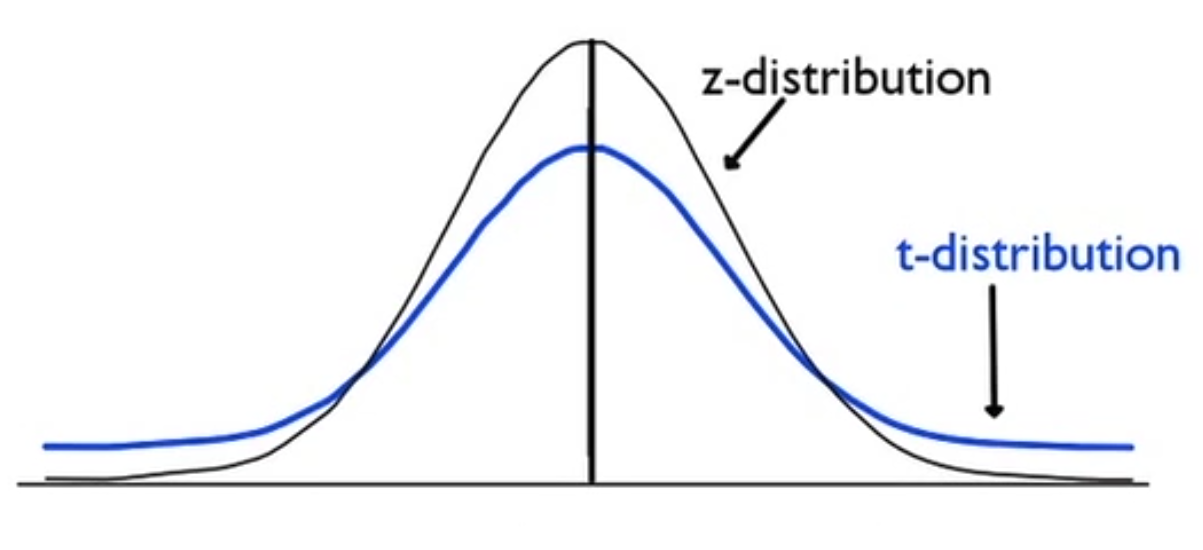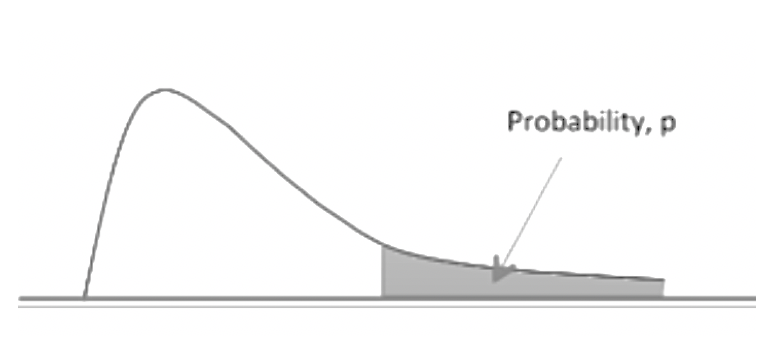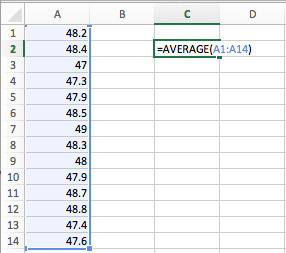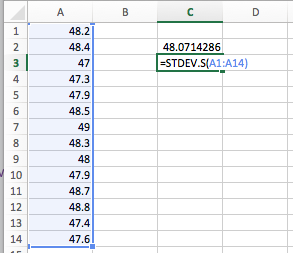+

# Calculating a T-Test Statistic

Author: Sophia Tutorial
##### Description:

Calculate a t-statistic of a sample mean.

(more)

Sophia’s self-paced online courses are a great way to save time and money as you earn credits eligible for transfer to many different colleges and universities.*

No credit card required

37 Sophia partners guarantee credit transfer.

299 Institutions have accepted or given pre-approval for credit transfer.

* The American Council on Education's College Credit Recommendation Service (ACE Credit®) has evaluated and recommended college credit for 33 of Sophia’s online courses. Many different colleges and universities consider ACE CREDIT recommendations in determining the applicability to their course and degree programs.

Tutorial
what's covered
In this tutorial, you will learn about t-tests, and how to determine key characteristics of a t-distribution. Our discussion breaks down as follows:

1. Difference between Z-Tests and T-Tests
2. Conducting a T-Test

## 1. Difference between Z-Tests and T-Tests

In a z-test for means, the z-test statistic is equal to the sample mean minus the hypothesized population mean, over the standard deviation of the population divided by the square root of sample size.

formula
Z-StatisticHowever, the z-statistic was based on the fact that the population standard deviation was known. If the population standard deviation is not known, we need a new statistic. We're going to use our sample standard deviation, s, instead.

formula
T-Statistichint
This "s" over the square root of n value, replacing the sigma over square root of n value, is called the standard error.

The only problem with using the sample standard deviation (s) as opposed to the population standard deviation (σ) is that the value of s can vary largely from sample to sample. Sigma (σ) is fixed, so we can base our normal distribution off of it.

The sample standard deviation is more variable than the population standard deviation and much more variable for small samples than for large samples. For large samples, s and sigma are very close, but with small samples particularly, the value of s can vary wildly.

Because s is so variable, it creates a new distribution of test statistics much like the normal distribution, but is known as the student's t-distribution, or sometimes just the t-distribution.The only difference is the t-distribution is a more heavy-tailed distribution. If we used the normal distribution, it wouldn't underestimate the proportion of extreme values in the sampling distribution.

The t-distribution is actually a family of distributions. They all are a little bit shorter than the standard normal distribution and a little heavier on the tails. As the sample size gets larger, the t-distribution does get close to the normal distribution. It doesn't diminish as quickly in the tails when the sample size is small, but gets very close to the normal distribution when n is large.

term to know

T-Distribution
A family of distributions that are centered at zero and symmetric like the standard normal distribution, but heavier in the tails. Depending on the sample size, it does not diminish towards the tails as fast. If the sample size is large, the t-distribution approximates the normal distribution.

## 2. Conducting a T-Test

We're going to conduct a t-test for population means much like we conducted a z-test for population means. Recall, that when running a hypothesis test, there are four parts:

step by step
1. State the null and alternative hypotheses.
2. Check the conditions necessary for inference.
3. Calculate the test statistic and its p-value.
4. Compare our test statistic to our chosen critical value, or our p-value, to the significance level, and then based on how those compare, make a decision about the null hypothesis and a conclusion in the context of the problem.

The only difference between these two tests is the test statistic is going to be a t-statistic instead of a z-statistic. Because we’re using the t-distribution instead of the z- distribution, we're going to obtain a different p-value.

Therefore, we will need a new table, not the standard normal table for that. Below is the t-distribution table. We can see the possible p-values in the top row and the t-values are the values inside the table. Potential p-values are based on the values within this section.

t-Distribution Critical Values
Tail Probability, p
One-tail 0.25 0.20 0.15 0.10 0.05 0.025 0.02 0.01 0.005 0.0025 0.001 0.0005
Two-tail 0.50 0.40 0.30 0.20 0.10 0.05 0.04 0.02 0.01 0.005 0.002 0.001
df
1 1.000 1.376 1.963 3.078 6.314 12.71 15.89 31.82 63.66 127.3 318.3 636.6
2 0.816 1.080 1.386 1.886 2.920 4.303 4.849 6.965 9.925 14.09 22.33 31.60
3 0.765 0.978 1.250 1.638 2.353 3.182 3.482 4.541 5.841 7.453 10.21 12.92
4 0.741 0.941 1.190 1.533 2.132 2.776 2.999 3.747 4.604 5.598 7.173 8.610
5 0.727 0.920 1.156 1.476 2.015 2.571 2.757 3.365 4.032 4.773 5.893 6.869
6 0.718 0.906 1.134 1.440 1.943 2.447 2.612 3.143 3.707 4.317 5.208 5.959
7 0.711 0.896 1.119 1.415 1.895 2.365 2.517 2.998 3.499 4.029 4.785 5.408
8 0.706 0.889 1.108 1.397 1.860 2.306 2.449 2.896 3.355 3.833 4.501 5.041
9 0.703 0.883 1.100 1.383 1.833 2.262 2.398 2.821 3.250 3.690 4.297 4.781
10 0.700 0.879 1.093 1.372 1.812 2.228 2.359 2.764 3.169 3.581 4.144 4.587
11 0.697 0.876 1.088 1.363 1.796 2.201 2.328 2.718 3.106 3.497 4.025 4.437
12 0.695 0.873 1.083 1.356 1.782 2.179 2.303 2.681 3.055 3.428 3.930 4.318
13 0.694 0.870 1.079 1.350 1.771 2.160 2.282 2.650 3.012 3.372 3.852 4.221
14 0.692 0.868 1.076 1.345 1.761 2.145 2.264 2.624 2.977 3.326 3.787 4.140
15 0.691 0.866 1.074 1.341 1.753 2.131 2.249 2.602 2.947 3.286 3.733 4.073
16 0.690 0.865 1.071 1.337 1.746 2.120 2.235 2.583 2.921 3.252 3.686 4.015
17 0.689 0.863 1.069 1.333 1.740 2.110 2.224 2.567 2.898 3.222 3.646 3.965
18 0.688 0.862 1.067 1.330 1.734 2.101 2.214 2.552 2.878 3.197 3.610 3.922
19 0.688 0.861 1.066 1.328 1.729 2.093 2.205 2.539 2.861 3.174 3.579 3.883
20 0.687 0.860 1.064 1.325 1.725 2.086 2.197 2.528 2.845 3.153 3.552 3.850
21 0.686 0.859 1.063 1.323 1.721 2.080 2.189 2.518 2.831 3.135 3.527 3.819
22 0.686 0.858 1.061 1.321 1.717 2.074 2.183 2.508 2.819 3.119 3.505 3.792
23 0.685 0.858 1.060 1.319 1.714 2.069 2.177 2.500 2.807 3.104 3.485 3.767
24 0.685 0.857 1.059 1.318 1.711 2.064 2.172 2.492 2.797 3.091 3.467 3.745
25 0.684 0.856 1.058 1.316 1.708 2.060 2.167 2.485 2.787 3.078 3.450 3.725
26 0.684 0.856 1.058 1.315 1.706 2.056 2.162 2.479 2.779 3.067 3.435 3.707
27 0.684 0.855 1.057 1.314 1.703 2.052 2.158 2.473 2.771 3.057 3.421 3.690
28 0.683 0.855 1.056 1.313 1.701 2.048 2.154 2.467 2.763 3.047 3.408 3.674
29 0.683 0.854 1.055 1.311 1.699 2.045 2.150 2.462 2.756 3.038 3.396 3.659
30 0.683 0.854 1.055 1.310 1.697 2.042 2.147 2.457 2.750 3.030 3.385 3.646
40 0.681 0.851 1.050 1.303 1.684 2.021 2.123 2.423 2.704 2.971 3.307 3.551
50 0.679 0.849 1.047 1.299 1.676 2.009 2.109 2.403 2.678 2.937 3.261 3.496
60 0.679 0.848 1.045 1.296 1.671 2.000 2.099 2.390 2.660 2.915 3.232 3.460
80 0.678 0.846 1.043 1.292 1.664 1.990 2.088 2.374 2.639 2.887 3.195 3.416
100 0.677 0.845 1.042 1.290 1.660 1.984 2.081 2.364 2.626 2.871 3.174 3.390
1000 0.675 0.842 1.037 1.282 1.646 1.962 2.056 2.330 2.581 2.813 3.098 3.300
>1000 0.674 0.841 1.036 1.282 1.645 1.960 2.054 2.326 2.576 2.807 3.091 3.291
Confidence Interval between -t and t
50% 60% 70% 80% 90% 95% 96% 98% 99% 99.5% 99.8% 99.9%

This distribution is actually one-sided, and it's the upper side that gives us these tail probabilities here. The entries in the t-table represent the probability of a value laying above t.The one new wrinkle that we're adding for a t-distribution is this value df (the far left column). It's called the degrees of freedom. For our purposes, it's going to be the sample size minus 1. We find our t-statistic in whatever row our degrees of freedom is. If it's between two values, that means our p-value is between these two p-values.

EXAMPLE

According to their bags, a standard bag of M&M's candies is supposed to weigh 47.9 grams. Suppose we randomly select 14 bags and got this distribution.

 48.2 48.4 47 47.3 47.9 48.5 49 48.3 48 47.9 48.7 48.8 47.4 47.6

Assuming the distribution of bag weights is approximately normal, is this evidence that the bags do not contain the amount of candy that they say that they do?
Step 1: State the null and alternative hypotheses.

The null is that the mean is 47.9 grams. The alternative is the mean is not 47.9 grams. We can select a significance level of 0.05, which means that if the p-value is less than 0.05, reject the null hypothesis.

• H0: μ = 47.9; The mean of all M&M's bags is 47.9g.
• Ha: μ ≠ 47.9; The mean of all M&M's bags is not 47.9g.
• α = 0.05
Step 2: Check the necessary conditions.

Consider the following criteria for a hypothesis test:

Criteria Description
Randomness How were the data collected?

The randomness should be stated somewhere in the problem. Think about the way the data was collected.
Independence Population ≥ 10n

You want to make sure that the population is at least 10 times as large as the sample size.
Normality n ≥ 30 or normal parent distribution

There are two ways to verify normality. Either the parent distribution has to be normal or the central limit theorem is going to have to apply. The central limit theorem says that for most distributions, when the sample size is greater than 30, the sampling distribution will be approximately normal.

Let's verify each of those in the M&M's problem:

• Randomness: It says in the problem that the bags were randomly selected.
• Independence: We're going to go ahead and assume that there are at least 140 bags of M&M's. That's 10 times as large as the 14 bags in our sample.
• Normality: Finally, it does say in the problem that the distribution of bag weights is approximately normal, and so normality will be verified for our sampling distribution.
Step 3: Calculate the test statistic and the p-value.

Since we do not know the population standard deviation, we will calculate a t-test. We first need to find the sample mean and standard deviation. We can easily find both values by using Excel. First, list all the values given. To find the average, type "=AVERAGE(" and highlight all 14 values. We can also find this function under the Formulas tab, and select the Math and Trigonometry option.When we press "Enter", we get an average of 48.07. We can also find the standard deviation easily by typing "=STDEV.S(" and highlighting the 14 values. We can also find this function by going under the Formulas tab, and then selecting the Statistical option.This gives a standard deviation of 0.60.

Now, we can plug the known values into the t-statistic formula:By plugging in all the numbers that we have, we obtain a t-statistic of positive 1.06. Where exactly does this tell us? We need to calculate the probability that we get a t-statistic of 1.06 or larger.

In the table, we also need to identify the degrees of freedom (df), which is the sample size minus one. The sample was 14 bags, so our degrees of freedom is 14 minus 1, or 13.

Let's look at the t-table in row 13 to find the closest value to 1.06:

t-Distribution Critical Values
Tail Probability, p
One-tail 0.25 0.20 0.15 0.10 0.05 0.025 0.02 0.01 0.005 0.0025 0.001 0.0005
Two-tail 0.50 0.40 0.30 0.20 0.10 0.05 0.04 0.02 0.01 0.005 0.002 0.001
df
1 1.000 1.376 1.963 3.078 6.314 12.71 15.89 31.82 63.66 127.3 318.3 636.6
2 0.816 1.080 1.386 1.886 2.920 4.303 4.849 6.965 9.925 14.09 22.33 31.60
3 0.765 0.978 1.250 1.638 2.353 3.182 3.482 4.541 5.841 7.453 10.21 12.92
4 0.741 0.941 1.190 1.533 2.132 2.776 2.999 3.747 4.604 5.598 7.173 8.610
5 0.727 0.920 1.156 1.476 2.015 2.571 2.757 3.365 4.032 4.773 5.893 6.869
6 0.718 0.906 1.134 1.440 1.943 2.447 2.612 3.143 3.707 4.317 5.208 5.959
7 0.711 0.896 1.119 1.415 1.895 2.365 2.517 2.998 3.499 4.029 4.785 5.408
8 0.706 0.889 1.108 1.397 1.860 2.306 2.449 2.896 3.355 3.833 4.501 5.041
9 0.703 0.883 1.100 1.383 1.833 2.262 2.398 2.821 3.250 3.690 4.297 4.781
10 0.700 0.879 1.093 1.372 1.812 2.228 2.359 2.764 3.169 3.581 4.144 4.587
11 0.697 0.876 1.088 1.363 1.796 2.201 2.328 2.718 3.106 3.497 4.025 4.437
12 0.695 0.873 1.083 1.356 1.782 2.179 2.303 2.681 3.055 3.428 3.930 4.318
13 0.694 0.870 1.079 1.350 1.771 2.160 2.282 2.650 3.012 3.372 3.852 4.221
14 0.692 0.868 1.076 1.345 1.761 2.145 2.264 2.624 2.977 3.326 3.787 4.140
15 0.691 0.866 1.074 1.341 1.753 2.131 2.249 2.602 2.947 3.286 3.733 4.073

In all likelihood, it's not one of the values listed in the row here, but between two values. We’ll see 1.06 is between the 0.870 and the 1.079, which means that the p-value is going to be between the two numbers 0.40 and 0.30 on the top row for a two-tailed test. Recall that we are testing to see if the mean weight of the M&M's bags is anything other than the hypothesized 47.9 grams, so this is a two-tailed test.

hint
You will need to consider if the problem is looking at a one-tailed or two-tailed test. If we need a value that is either less than or greater than the hypothesized mean, we will use a one-tailed test. If we are looking for any value that is different than the hypothesized mean, then we will use a two-tailed test.

Now, we can, in fact, use technology to nail down the p-value more exactly. We don't have to use this table, although we can still use the table to answer the question about the null hypothesis.

Step 4: Compare our p-value to our significance level.

We don't know exactly what our p-value is, but we know that it's within the range of 0.30 to 0.40. Since both of those numbers is greater than the significance level of 0.05, we're going to fail to reject the null hypothesis. There's our decision based on how they compare.

Finally, the conclusion is that there's not sufficient evidence to conclude that M&M's bags are being filled to a mean other than 47.9 grams.

term to know

T-test For Population Means
The type of hypothesis test used to test an assumed population mean when the population standard deviation is unknown. Due to the increased variability in using the sample standard deviation instead of the population standard deviation, the t-distribution is used in place of the z-distribution.

summary
In cases where the population standard deviation is not known--which is almost always--we should use the t-distribution to account for the additional variability introduced by using the sample standard deviation in the test statistic. A t-test means that the value will be a "t" statistic instead of a "z" statistic. The steps in the hypothesis test are the same as they are in a z-test: first stating the non-alternative hypotheses, stating and verifying the conclusions of the test, calculating the test statistic and the p-value, and then finally, comparing the p-value to alpha and making a decision about the null hypothesis.

Good luck!

Source: Adapted from Sophia tutorial by Jonathan Osters.

## T-Table

of
Terms to Know
T-Distribution/Student's T-Distribution

A family of distributions that are centered at zero and symmetric like the standard normal distribution, but heavier in the tails. Depending on the sample size, it does not diminish towards the tails as fast. If the sample size is large, the t-distribution approximates the normal distribution.

T-test For Population Means

The type of hypothesis test used to test an assumed population mean when the population standard deviation is unknown. Due to the increased variability in using the sample standard deviation instead of the population standard deviation, the t-distribution is used in place of the z-distribution.

Formulas to Know
T-Statistic For Population MeansRating